# Understanding Differential Equations Chapter Exam

Exam Instructions:

Choose your answers to the questions and click 'Next' to see the next set of questions. You can skip questions if you would like and come back to them later with the yellow "Go To First Skipped Question" button. When you have completed the practice exam, a green submit button will appear. Click it to see your results. Good luck!

### Page 1

#### Question 1 1. Solve the following differential equation for y=f(x) (assume that y is always positive).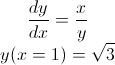#### Question 2 2. Solve the following differential equation for c=f(a).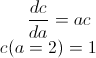#### Question 3 3. Solve the following differential equation for y=f(x).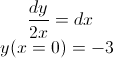#### Question 4 4. Solve the following differential equation for y=f(x) (assume that y is always positive).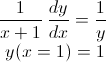#### Question 5 5. Solve the following differential equation for y=f(x).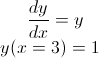### Page 3

#### Understanding Differential Equations Chapter Exam Instructions

Choose your answers to the questions and click 'Next' to see the next set of questions. You can skip questions if you would like and come back to them later with the yellow "Go To First Skipped Question" button. When you have completed the practice exam, a green submit button will appear. Click it to see your results. Good luck!

Support